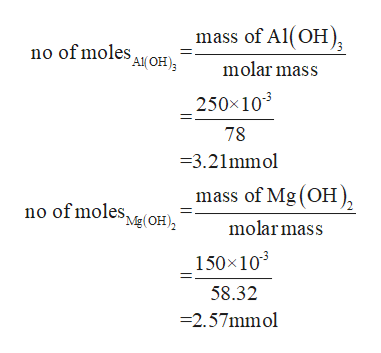# Calculate the maximum volume in mL of 0.15 M HCl that each of the following antacid formulations would be expected to neutralize. Assume complete neutralization. (Show work.) a) a tablet containing 250 mg Al(OH)3 and 150 mg Mg(OH)2b) a tablet containing 850 mg of CaCO3

Question
16 views

Calculate the maximum volume in mL of 0.15 M HCl that each of the following antacid formulations would be expected to neutralize. Assume complete neutralization. (Show work.)
a) a tablet containing 250 mg Al(OH)3 and 150 mg Mg(OH)2

b) a tablet containing 850 mg of CaCO3

check_circle

Step 1

Given

Mass of Al(OH)3 = 250mg

Mass of Mg(OH)2 = 150mg

Mass of CaCO3 = 850mg

Molarity of HCl= 0.15M

The reactions with the given compounds are

Step 2

No of moleshelp_outlineImage Transcriptionclosemass of Al(OH 'A(OH) no of moles molar mass 250x103 78 =3.21mmol mass of Mg (OH no of moles Mg(OH) molarmass 150x103 58.32 -2.57mmol fullscreen
Step 3

According to the reactions

3 moles of HCl are required per mole of Al(OH)3 and 2 moles of HCl are re...

### Want to see the full answer?

See Solution

#### Want to see this answer and more?

Solutions are written by subject experts who are available 24/7. Questions are typically answered within 1 hour.*

See Solution
*Response times may vary by subject and question.
Tagged in

### Chemistry